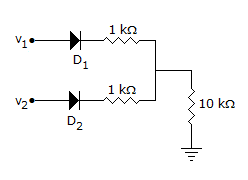# Anubhava Circuit Design Challenge

50 Questions | Total Attempts: 303SettingsINSTRUCTIONS:Every question contains negative points (+2 for correct, -1 for wrong)Please submit before time runs out. Team members can be 2-3. Once team is formed cannot be changedALL THE BEST!

• 1.
A 33 half-watt resistor and a 330 half-watt resistor are connected across a 12 V source. Which one(s) will overheat?
• A.

33

• B.

330

• C.

Both resistors

• D.

Neither resistors

• 2.
When the current through the coil of an electromagnet reverses, the
• A.

direction of the magnetic field reverses

• B.

Direction of the magnetic field remains unchanged

• C.

Magnetic field expands

• D.

magnetic field collapses

• 3.
When a 15 V input pulse with a width equal to two time constants is applied to an RC integrator, the capacitor charges to
• A.

15

• B.

12.9

• C.

8.6

• D.

19.45

• 4.
If all the elements in a particular network are linear, then the superposition theorem would hold, when the excitation is
• A.

DC only

• B.

AC only

• C.

Either AC or DC

• D.

An Impulse

• 5.
Mesh analysis is applicable for
• A.

Planar networks

• B.

Non planar networks

• C.

Both planner and non-planar networks.

• D.

Neither planner nor non planner networks

• 6.
Which of the following memories uses one transistor and one capacitor as basic memory unit
• A.

SRAM

• B.

DRAM

• C.

Both SRAM and DRAM

• D.

Neither of them

• 7.
A external resistance R is connected to a cell of internal resistance r, then the current is maximum when
• A.

R>r

• B.

R < r

• C.

R=r

• D.

None

• 8.
What is referred as the average value in AC operation?
• A.

TriangleAverage of all values of an alternating quantity.

• B.

Average of all values of the phase sequences.

• C.

Average of all values of the (+)ve and (-)ve half.

• D.

Average of all values of an alternating quantity over a complete cycle.

• 9.
An electric field line and an equipotential surface are
• A.

Always 90°.

• B.

Always parallel.

• C.

Inclined at any angle.

• D.

None

• 10.
For a collpits oscillator, the capacitor values are 1micro farad and 10 micro farad. The inductor is 10 henry .Its frequency of oscillation approximately is,
• A.

400Hz

• B.

50Hz

• C.

1Hz

• D.

100Hz

• 11.
Among the following the audio frequency oscillators are 1)rc phase shift 2)weins bridge 3) Hartley 4) collpit
• A.

1 and 2

• B.

3 and 4

• C.

1 and 3

• D.

1 and 4

• 12.
The amplifiers used in radio and tv transmitters are
• A.

Class A

• B.

Class B

• C.

Class AB

• D.

Class C

• 13.
In a transistor which is moderately doped
• A.

Collector

• B.

Emitter

• C.

Base

• D.

None

• 14.
Generally,the configuration used in high frequency circuits is
• A.

Common base

• B.

Common Collector

• C.

Common Emitter

• D.

None

• 15.
Generally,the configuration used in impedence matching is,
• A.

Common base

• B.

Common Collector

• C.

Common Emitter

• D.

None

• 16.
In a voltage series connection the input impedence when compared to normal amplifier is
• A.

Decreases

• B.

Increases

• C.

No change

• D.

Can't determined

• 17.
Commutative property of or gate is
• A.

A+B=B+A

• B.

AB=BA

• C.

A(B+C)=AB+AC

• D.

A+BC=(A+B)(A+C)

• 18.
Which of the following is a digital device
• A.

Regulator of a fan

• B.

Microphone

• C.

Resistance of a material

• D.

Light switch

• 19.
Which logic family provide minimum power dissipation
• A.

TTL

• B.

CMOS

• C.

ECL

• D.

JFET

• 20.
Which is the example of digital device from the given option ?
• A.

Sensors

• B.

Recorder players

• C.

Microprocessors

• D.

Thermistors

• 21.
NPN transistor is not suitable for good analog switch because
• A.

IC −VCE characteristic curve pass directly through origin

• B.

The device has very high input impedance.

• C.

The device is asymmetrical with an offset voltage VCE off.

• D.

It has well defined transition frequency

• 22.
In figure v1 = 8 V and v2 = 4 V. Which diode will conduct?
• A.

Only D1

• B.

Only D2

• C.

Both D1 and D2

• D.

None

• 23.
Simplification following Boolean expression gives, Expression= (A'BC')'+(AB'C)'
• A.

1

• B.

A

• C.

Bc

• D.

0

• 24.
The most commonly used amplifier in sample and hold circuit is
• A.

A unity gain inverting amplifier

• B.

A unity gain non inverting amplifier

• C.

An inverting amplifier with a gain of 10

• D.

An inverting amplifier with a gain of 100

• 25.
When the ac base voltage in a CE amplifier circuit is too high, the ac emitter current is
• A.

Zero

• B.

Constant

• C.

Alternating

• D.

Distorted

Related TopicsBack to top